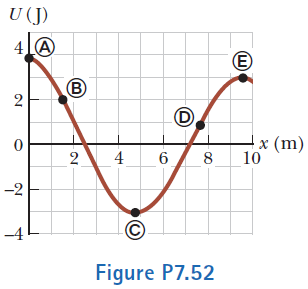# Problem: For the potential energy curve shown in Figure P7.52, (a) determine whether the force Fx is positive, negative, or zero at the five points indicated. (b) Indicate points of stable, unstable, and neutral equilibrium. (c) Sketch the curve for Fx versus x from x = 0 to x = 9.5 m.

###### FREE Expert Solution
92% (230 ratings)
###### FREE Expert Solution

in this problem, we'll apply the relationship between Force, potential, and the change in position.

Potential energy:

92% (230 ratings)###### Problem Details

For the potential energy curve shown in Figure P7.52, (a) determine whether the force Fx is positive, negative, or zero at the five points indicated. (b) Indicate points of stable, unstable, and neutral equilibrium. (c) Sketch the curve for Fx versus x from x = 0 to x = 9.5 m.Frequently Asked Questions

What scientific concept do you need to know in order to solve this problem?

Our tutors have indicated that to solve this problem you will need to apply the Force & Potential Energy concept. You can view video lessons to learn Force & Potential Energy. Or if you need more Force & Potential Energy practice, you can also practice Force & Potential Energy practice problems.

What professor is this problem relevant for?

Based on our data, we think this problem is relevant for Professor Tennant's class at SFSU.

What textbook is this problem found in?

Our data indicates that this problem or a close variation was asked in Physics for Scientists and Engineers - Serway Calc 9th Edition. You can also practice Physics for Scientists and Engineers - Serway Calc 9th Edition practice problems.